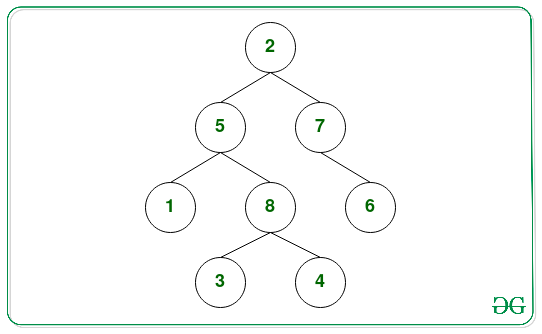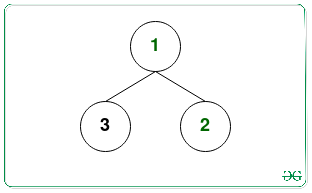GFG App
Open AppBrowser
Continue

# Count of leaf nodes required to be removed at each step to empty a given Binary Tree

Given a Binary Tree, the task is to remove the leaf nodes of the Binary Tree during each operation and print the count.

Examples:

Input:Output: 4 2 1 1
Explanation:
In the 1st operation removing the leaf nodes { 1, 3, 4, 6 } from the binary tree.
In the 2nd operation removing the leaf nodes { 8, 7 }
In the 3rd operation removing the leaf nodes { 5 }
In the 4th operation removing the leaf nodes { 2 }
Therefore, the count of leaf nodes removed in each operation 4 2 1 1.

Input:Output: 2 1

## Recommended: Please solve it on “PRACTICE” first, before moving on to the solution.

Naive Approach: The simplest approach to solve this problem to repeatedly traverse the tree for each operation and print the count of leaf nodes currently present in the binary tree and delete all the leaf nodes from the binary tree

Time Complexity: O(N2)
Auxiliary Space: O(1)

Efficient Approach: To optimize the above approach, the idea is to observe that, a node is not deleted unless both its children have been deleted already. Therefore, it can be determined that on which step a node will be deleted, which will be equal to 1 + maximum path length from that node to any leaf node in the subtree rooted at that node. Follow the steps below to solve this problem:

• Initialize a Map, say M, to store the leaf nodes at each deletion from the tree.
• Perform a DFS Traversal on the given Binary Tree following the steps below:
• Check if the given node is NULL or not. If found to be true, then return 0.
• Otherwise, recursively call for left and right nodes and store the values returned from it.
• Find the maximum height, say maxHeight, when each node will be a leaf node as (1 + maximum of the values returned by the left and right recursive calls) in the above steps for each node.
• Insert the current node in the Map M at key maxHeight.
• After completing the above steps, print the count of leaf nodes stored in the Map after each deletion.

Below is the implementation of the above approach:

## C++

 `// C++ program for the above approach`   `#include ` `using` `namespace` `std;`   `// Structure of a` `// Binary Tree Node` `struct` `Node {` `    ``int` `data;` `    ``Node *left, *right;` `};`   `// Function to allocate` `// a new tree node` `Node* newNode(``int` `data)` `{` `    ``Node* node = ``new` `Node;` `    ``node->data = data;` `    ``node->left = NULL;` `    ``node->right = NULL;`   `    ``return` `node;` `}`   `// Function to find the maximum height` `// from leaf in the subtree rooted at` `// current node and add that to hashmap` `int` `maxHeightToLeafUTIL(` `    ``Node* curr, map<``int``, vector<``int``> >& mp)` `{` `    ``if` `(curr == NULL) {` `        ``return` `0;` `    ``}`   `    ``// Max height to leaf in left subtree` `    ``int` `leftLeaf` `        ``= maxHeightToLeafUTIL(curr->left, mp);`   `    ``// Max height to leaf in right subtree` `    ``int` `rightLeaf` `        ``= maxHeightToLeafUTIL(curr->right, mp);`   `    ``// Max height to leaf in current subtree` `    ``int` `maxHeightSubtree` `        ``= 1 + max(leftLeaf, rightLeaf);`   `    ``// Adding current node to the Map` `    ``mp[maxHeightSubtree].push_back(` `        ``curr->data);`   `    ``return` `maxHeightSubtree;` `}`   `// Function to find the count of leaf nodes` `// by repeatedly removing the leaf nodes` `void` `printAndDelete(Node* root)` `{`   `    ``// Stores the leaf deletion with` `    ``// each iteration` `    ``map<``int``, vector<``int``> > mp;`   `    ``// Function Call to find the order` `    ``// of deletion of nodes` `    ``maxHeightToLeafUTIL(root, mp);`   `    ``// Printing the map values` `    ``for` `(``auto` `step : mp) {`   `        ``cout << mp[step.first].size() << ``" "``;` `    ``}` `}`   `// Driver code` `int` `main()` `{` `    ``// Given Binary Tree` `    ``Node* root = newNode(2);` `    ``root->right = newNode(7);` `    ``root->right->right = newNode(6);` `    ``root->left = newNode(5);` `    ``root->left->left = newNode(1);` `    ``root->left->right = newNode(8);` `    ``root->left->right->left = newNode(3);` `    ``root->left->right->right = newNode(4);`   `    ``/*` `    ``Input :` `         ``2` `       ``/   \` `      ``5     7` `     ``/ \     \` `    ``1   8     6` `       ``/ \` `      ``3   4` `*/`   `    ``// Function Call` `    ``printAndDelete(root);`   `    ``return` `0;` `}`   `// This code is contributed by pragup`

## Java

 `// Java program for the above approach` `import` `java.util.*;`   `// A binary tree node` `class` `Node ` `{` `  ``int` `data;` `  ``Node left, right;` `  ``Node(``int` `item) ` `  ``{` `    ``data = item;` `    ``left = right = ``null``;` `  ``}` `}`   `class` `GFG ` `{` `  ``Node root;`   `  ``// Function to find the maximum height` `  ``// from leaf in the subtree rooted at` `  ``// current node and add that to hashmap` `  ``static` `int` `maxHeightToLeafUTIL(` `    ``Node curr, Map > mp)` `  ``{` `    ``if` `(curr == ``null``)` `    ``{` `      ``return` `0``;` `    ``}`   `    ``// Max height to leaf in left subtree` `    ``int` `leftLeaf` `      ``= maxHeightToLeafUTIL(curr.left, mp);`   `    ``// Max height to leaf in right subtree` `    ``int` `rightLeaf` `      ``= maxHeightToLeafUTIL(curr.right, mp);`   `    ``// Max height to leaf in current subtree` `    ``int` `maxHeightSubtree` `      ``= ``1` `+ Math.max(leftLeaf, rightLeaf);`   `    ``// Adding current node to the Map` `    ``if``(!mp.containsKey(maxHeightSubtree))` `    ``{` `      ``mp.put(maxHeightSubtree, ``new` `ArrayList<>());   ` `    ``}`   `    ``mp.get(maxHeightSubtree).add(curr.data);` `    ``return` `maxHeightSubtree;` `  ``}`   `  ``// Function to find the count of leaf nodes` `  ``// by repeatedly removing the leaf nodes` `  ``static` `void` `printAndDelete(Node root)` `  ``{`   `    ``// Stores the leaf deletion with` `    ``// each iteration` `    ``Map > mp=``new` `HashMap<>();`   `    ``// Function Call to find the order` `    ``// of deletion of nodes` `    ``maxHeightToLeafUTIL(root, mp);`   `    ``// Printing the map values` `    ``for` `(Map.Entry> k:mp.entrySet()) ` `    ``{` `      ``System.out.print(k.getValue().size() + ``" "``);` `    ``}` `  ``}`   `  ``// Driver code` `  ``public` `static` `void` `main (String[] args) ` `  ``{`   `    ``GFG tree = ``new` `GFG();`   `    ``tree.root = ``new` `Node(``2``);` `    ``tree.root.left = ``new` `Node(``5``);` `    ``tree.root.right = ``new` `Node(``7``);` `    ``tree.root.right.right = ``new` `Node(``6``);` `    ``tree.root.left.left = ``new` `Node(``1``);` `    ``tree.root.left.right = ``new` `Node(``8``);` `    ``tree.root.left.right.left = ``new` `Node(``3``);` `    ``tree.root.left.right.right = ``new` `Node(``4``);`   `    ``/*` `    ``Input :` `         ``2` `       ``/   \` `      ``5     7` `     ``/ \     \` `    ``1   8     6` `       ``/ \` `      ``3   4` `*/`   `    ``// Function Call` `    ``printAndDelete(tree.root);` `  ``}` `}`   `// This code is contributed by offbeat`

## Python3

 `# Python3 program for the above approach `   `# Tree node structure used in the program` `class` `Node:` `    `  `    ``def` `__init__(``self``, x):` `        `  `        ``self``.data ``=` `x` `        ``self``.left ``=` `None` `        ``self``.right ``=` `None`   `# Function to find the maximum height` `# from leaf in the subtree rooted at` `# current node and add that to hashmap` `def` `maxHeightToLeafUTIL(curr):` `    `  `    ``global` `mp`   `    ``if` `(curr ``=``=` `None``):` `        ``return` `0`   `    ``# Max height to leaf in left subtree` `    ``leftLeaf ``=` `maxHeightToLeafUTIL(curr.left)`   `    ``# Max height to leaf in right subtree` `    ``rightLeaf ``=` `maxHeightToLeafUTIL(curr.right)`   `    ``# Max height to leaf in current subtree` `    ``maxHeightSubtree ``=` `1` `+` `max``(leftLeaf, rightLeaf)`   `    ``# Adding current node to the Map` `    ``mp[maxHeightSubtree].append(curr.data)`   `    ``return` `maxHeightSubtree`   `# Function to find the count of leaf nodes` `# by repeatedly removing the leaf nodes` `def` `printAndDelete(root):` `    `  `    ``global` `mp` `    `  `    ``# Function Call to find the order` `    ``# of deletion of nodes` `    ``maxHeightToLeafUTIL(root)`   `    ``for` `step ``in` `mp:` `        ``if` `len``(step):` `            ``print``(``len``(step), end ``=` `" "``)`   `# Driver code` `if` `__name__ ``=``=` `'__main__'``:` `    `  `    ``mp ``=` `[[] ``for` `i ``in` `range``(``1000``)]` `    `  `    ``# Given Binary Tree` `    ``root ``=` `Node(``2``)` `    ``root.right ``=` `Node(``7``)` `    ``root.right.right ``=` `Node(``6``)` `    ``root.left ``=` `Node(``5``)` `    ``root.left.left ``=` `Node(``1``)` `    ``root.left.right ``=` `Node(``8``)` `    ``root.left.right.left ``=` `Node(``3``)` `    ``root.left.right.right ``=` `Node(``4``)` `    ``#` `    ``#     ` `    ``#     Input :` `    ``#          2` `    ``#        /   \` `    ``#       5     7` `    ``#      / \     \` `    ``#     1   8     6` `    ``#        / \` `    ``#       3   4`   `    ``# Function Call` `    ``printAndDelete(root)` `    `  `# This code is contributed by mohit kumar 29`

## C#

 `// C# program for the above approach` `using` `System;` `using` `System.Collections.Generic;`   `// A binary tree node` `public` `class` `Node` `{` `  ``public` `int` `data;` `  ``public` `Node left, right;` `  ``public` `Node(``int` `item)` `  ``{` `    ``data = item;` `    ``left = right = ``null``;` `  ``}` `}`   `public` `class` `GFG{` `    `  `    ``Node root;` ` `  `  ``// Function to find the maximum height` `  ``// from leaf in the subtree rooted at` `  ``// current node and add that to hashmap` `  ``static` `int` `maxHeightToLeafUTIL(` `    ``Node curr, Dictionary<``int``, List<``int``> > mp)` `  ``{` `    ``if` `(curr == ``null``)` `    ``{` `      ``return` `0;` `    ``}` ` `  `    ``// Max height to leaf in left subtree` `    ``int` `leftLeaf` `      ``= maxHeightToLeafUTIL(curr.left, mp);` ` `  `    ``// Max height to leaf in right subtree` `    ``int` `rightLeaf` `      ``= maxHeightToLeafUTIL(curr.right, mp);` ` `  `    ``// Max height to leaf in current subtree` `    ``int` `maxHeightSubtree` `      ``= 1 + Math.Max(leftLeaf, rightLeaf);` ` `  `    ``// Adding current node to the Map` `    ``if``(!mp.ContainsKey(maxHeightSubtree))` `    ``{` `      ``mp.Add(maxHeightSubtree, ``new` `List<``int``>());  ` `    ``}` ` `  `    ``mp[maxHeightSubtree].Add(curr.data);` `    ``return` `maxHeightSubtree;` `  ``}` ` `  `  ``// Function to find the count of leaf nodes` `  ``// by repeatedly removing the leaf nodes` `  ``static` `void` `printAndDelete(Node root)` `  ``{` ` `  `    ``// Stores the leaf deletion with` `    ``// each iteration` `    ``Dictionary<``int``, List<``int``> > mp=``new` `Dictionary<``int``, List<``int``> >();` ` `  `    ``// Function Call to find the order` `    ``// of deletion of nodes` `    ``maxHeightToLeafUTIL(root, mp);` ` `  `    ``// Printing the map values` `    ``foreach``(KeyValuePair<``int``, List<``int``>> k ``in` `mp)` `    ``{` `      ``Console.Write(k.Value.Count + ``" "``);` `    ``}` `  ``}` ` `  `  ``// Driver code` `    `  `    ``static` `public` `void` `Main (){` `        `  `        ``GFG tree = ``new` `GFG();` ` `  `    ``tree.root = ``new` `Node(2);` `    ``tree.root.left = ``new` `Node(5);` `    ``tree.root.right = ``new` `Node(7);` `    ``tree.root.right.right = ``new` `Node(6);` `    ``tree.root.left.left = ``new` `Node(1);` `    ``tree.root.left.right = ``new` `Node(8);` `    ``tree.root.left.right.left = ``new` `Node(3);` `    ``tree.root.left.right.right = ``new` `Node(4);` ` `  `    ``/*` `    ``Input :` `         ``2` `       ``/   \` `      ``5     7` `     ``/ \     \` `    ``1   8     6` `       ``/ \` `      ``3   4` `*/` ` `  `    ``// Function Call` `    ``printAndDelete(tree.root);` `    ``}` `}`

## Javascript

 ``

Output:

`4 2 1 1`

Time Complexity: O(N)
Auxiliary Space: O(N)

My Personal Notes arrow_drop_up